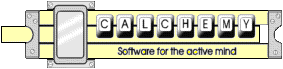# Quantity Equations

### Quantity equations relate the physical properties of measured quantities.

Quantity equations are the explanations of physics that relate the dimensions of Length, mass, time, temperature and substance. These equations have been developed through a long history of scientific study and discovery. The relationships described by quantity equations result directly from basic physical "laws", such as Newtonian mechanics the conservation of energy and momentum, the geometric definitions of area, volume density etc.

Quantity equations do not specify that the input parameters have specific units. Instead, quantity equations may be solved using any coherent system of units.

What Calchemy does is coherse the various systems of units into being mutually coherent! Calchemy allows you to enter the parameters of quantity equations in whatever units you know them in, and gives you the result in whatever units you want.

In practice, it has been common to employ equations that require the use of convenient or familiar units of measure to represent the variables. In contrast to Quantity Equations, these so called "numeric" or "engineering" equations contain some conversion factor that represents the combined conversions required to make the units of the equation coherent. These conversion factors are often dimensionless, but in fact do have units (like ft/meter). This (misleading) representation results in a non-coherent equation.

### Calchemy cannot be used to solve non-coherent "numeric" or "engineering" equations.

Calchemy Software Inc.
Fort Collins, COLast Updated 6/21/2009